# Tag: Kapranov

One of the more surprising analogies around is that prime numbers can be viewed as knots in the 3-sphere $S^3$. The motivation behind it is that the (etale) fundamental group of $\pmb{spec}(\mathbb{Z}/(p))$ is equal to (the completion) of the fundamental group of a circle $S^1$ and that the embedding

$\pmb{spec}(\mathbb{Z}/(p)) \subset \pmb{spec}(\mathbb{Z})$

embeds this circle as a knot in a 3-dimensional simply connected manifold which, after Perelman, has to be $S^3$. For more see the what is the knot associated to a prime?-post.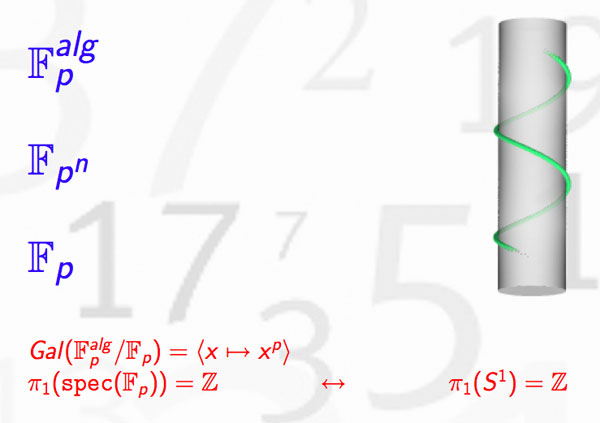In recent months new evidence has come to light allowing us to settle the genesis of this marvelous idea.

1. The former consensusUntil now, the generally accepted view (see for example the ‘Mazur-dictionary-post’ or Morishita’s expository paper) was that the analogy between knots and primes was first pointed out by Barry Mazur in the middle of the 1960’s when preparing for his lectures at the Summer Conference on Algebraic Geometry, at Bowdoin, in 1966. The lecture notes where later published in 1973 in the Annales of the ENS as ‘Notes on etale cohomology of number fields’.

For further use in this series of posts, please note the acknowledgement at the bottom of the first page, reproduced below : “It gives me pleasure to thank J.-P. Serre for his vigorous editing and his suggestions and corrections, which led to this revised version.”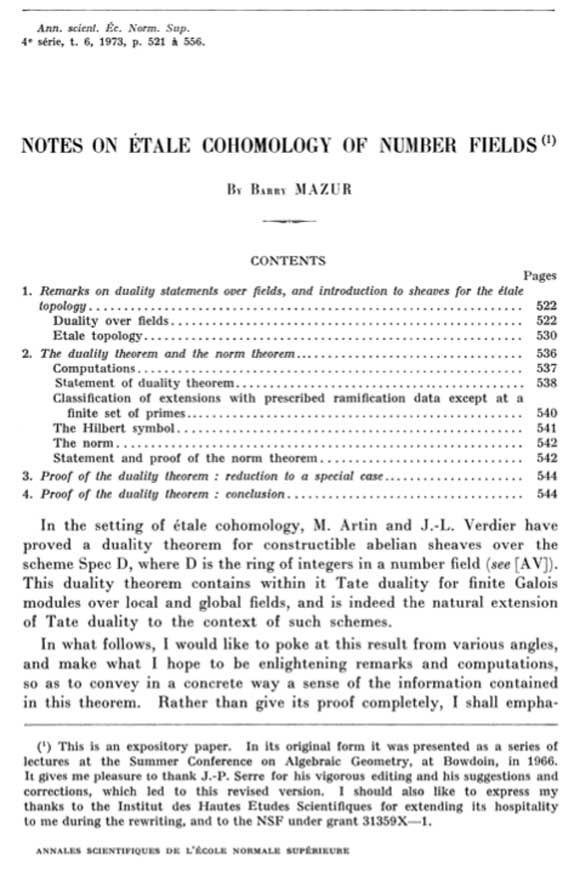Independently, Yuri I. Manin spotted the same analogy at around the same time. However, this point of view was quickly forgotten in favor of the more classical one of viewing number fields as analogous to algebraic function fields of one variable. Subsequently, in the mid 1990’s Mikhail Kapranov and Alexander Reznikov took up the analogy between number fields and 3-manifolds again, and called the resulting study arithmetic topology.

2. The new evidence

On december 13th 2010, David Feldman posted a MathOverflow-question Mazur’s unpublished manuscript on primes and knots?. He wrote : “The story of the analogy between knots and primes, which now has a literature, started with an unpublished note by Barry Mazur. I’m not absolutely sure this is the one I mean, but in his paper, Analogies between group actions on 3-manifolds and number fields, Adam Sikora cites B. Mazur, Remarks on the Alexander polynomial, unpublished notes.

Two months later, on february 15th David Feldman suddenly found the missing preprint in his mail-box and made it available. The preprint is now also available from Barry Mazur’s website. Mazur adds the following comment :

“In 1963 or 1964 I wrote an article Remarks on the Alexander Polynomial [PDF] about the analogy between knots in the three-dimensional sphere and prime numbers (and, correspondingly, the relationship between the Alexander polynomial and Iwasawa Theory). I distributed some copies of my article but never published it, and I misplaced my own copy. In subsequent years I have had many requests for my article and would often try to search through my files to find it, but never did. A few weeks ago Minh-Tri Do asked me for my article, and when I said I had none, he very kindly went on the web and magically found a scanned copy of it. I’m extremely grateful to Minh-Tri Do for his efforts (and many thanks, too, to David Feldman who provided the lead).”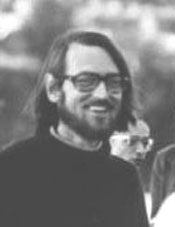The opening paragraph of this unpublished preprint contains a major surprise!

Mazur points to David Mumford as the originator of the ‘primes-are-knots’ idea : “Mumford has suggested a most elegant model as a geometric interpretation of the above situation : $\pmb{spec}(\mathbb{Z}/p\mathbb{Z})$ is like a one-dimensional knot in $\pmb{spec}(\mathbb{Z})$ which is like a simply connected three-manifold.”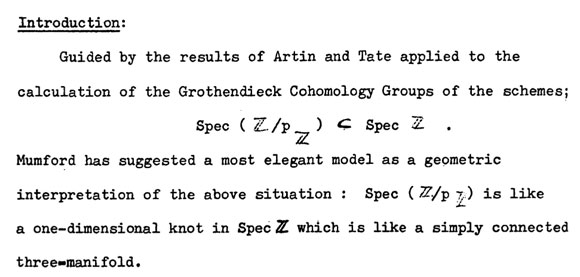In a later post we will show that one can even pinpoint the time and place when and where this analogy was first dreamed-up to within a few days and a couple of miles.

For the impatient among you, have a sneak preview of the cradle of birth of the primes=knots idea…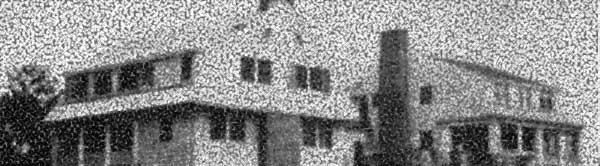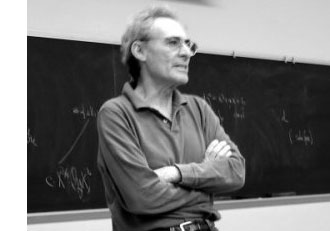In the previous posts, we have depicted the ‘arithmetic line’, that is the prime numbers, as a ‘line’ and individual primes as ‘points’.

However, sometime in the roaring 60-ties, Barry Mazur launched the crazy idea of viewing the affine spectrum of the integers, $\mathbf{spec}(\mathbb{Z})$, as a 3-dimensional manifold and prime numbers themselves as knots in this 3-manifold…

After a long silence, this idea was taken up recently by Mikhail Kapranov and Alexander Reznikov (1960-2003) in a talk at the MPI-Bonn in august 1996. Pieter Moree tells the story in his recollections about Alexander (Sacha) Reznikov in Sipping Tea with Sacha : “Sasha’s paper is closely related to his paper where the analogy of covers of three-manifolds and class field theory plays a big role (an analogy that was apparently first noticed by B. Mazur). Sasha and Mikhail Kapranov (at the time also at the institute) were both very interested in this analogy. Eventually, in August 1996, Kapranov and Reznikov both lectured on this (and I explained in about 10 minutes my contribution to Reznikov’s proof). I was pleased to learn some time ago that this lecture series even made it into the literature, see Morishita’s ‘On certain analogies between knots and primes’ J. reine angew. Math 550 (2002) 141-167.”

Here’s a part of what is now called the Kapranov-Reznikov-Mazur dictionary :What is the rationale behind this dictionary? Well, it all has to do with trying to make sense of the (algebraic) fundamental group $\pi_1^{alg}(X)$ of a general scheme $X$. Recall that for a manifold $M$ there are two different ways to define its fundamental group $\pi_1(M)$ : either as the closed loops in a given basepoint upto homotopy or as the automorphism group of the universal cover $\tilde{M}$ of $M$.

For an arbitrary scheme the first definition doesn’t make sense but we can use the second one as we have a good notion of a (finite) cover : an etale morphism $Y \rightarrow X$ of the scheme $X$. As they form an inverse system, we can take their finite automorphism groups $Aut_X(Y)$ and take their projective limit along the system and call this the algebraic fundamental group $\pi^{alg}_1(X)$.

Hendrik Lenstra has written beautiful course notes on ‘Galois theory for schemes’ on all of this starting from scratch. Besides, there are also two video-lectures available on this at the MSRI-website : Etale fundamental groups 1 by H.W. Lenstra and Etale fundamental groups 2 by F. Pop.

But, what is the connection with the ‘usual’ fundamental group in case both of them can be defined? Well, by construction the algebraic fundamental group is always a profinite group and in the case of manifolds it coincides with the profinite completion of the standard fundamental group, that is,
$\pi^{alg}_1(M) \simeq \widehat{\pi_1(M)}$ (recall that the cofinite completion is the projective limit of all finite group quotients).

Right, so all we have to do to find a topological equivalent of an algebraic scheme is to compute its algebraic fundamental group and find an existing topological space of which the profinite completion of its standard fundamental group coincides with our algebraic fundamental group. An example : a prime number $p$ (as a ‘point’ in $\mathbf{spec}(\mathbb{Z})$) is the closed subscheme $\mathbf{spec}(\mathbb{F}_p)$ corresponding to the finite field $\mathbb{F}_p = \mathbb{Z}/p\mathbb{Z}$. For any affine scheme of a field $K$, the algebraic fundamental group coincides with the absolute Galois group $Gal(\overline{K}/K)$. In the case of $\mathbb{F}_p$ we all know that this abslute Galois group is isomorphic with the profinite integers $\hat{\mathbb{Z}}$. Now, what is the first topological space coming to mind having the integers as its fundamental group? Right, the circle $S^1$. Hence, in arithmetic topology we view prime numbers as topological circles, that is, as knots in some bigger space.

But then, what is this bigger space? That is, what is the topological equivalent of $\mathbf{spec}(\mathbb{Z})$? For this we have to go back to Mazur’s original paper Notes on etale cohomology of number fields in which he gives an Artin-Verdier type duality theorem for the affine spectrum $X=\mathbf{spec}(D)$ of the ring of integers $D$ in a number field. More precisely, there is a non-degenerate pairing $H^r_{et}(X,F) \times Ext^{3-r}_X(F, \mathbb{G}_m) \rightarrow H^3_{et}(X,F) \simeq \mathbb{Q}/\mathbb{Z}$ for any constructible abelian sheaf $F$. This may not tell you much, but it is a ‘sort of’ Poincare-duality result one would have for a compact three dimensional manifold.

Ok, so in particular $\mathbf{spec}(\mathbb{Z})$ should be thought of as a 3-dimensional compact manifold, but which one? For this we have to compute the algebraic fundamental group. Fortunately, this group is trivial as there are no (non-split) etale covers of $\mathbf{spec}(\mathbb{Z})$, so the corresponding 3-manifold should be simple connected… but wenow know that this has to imply that the manifold must be $S^3$, the 3-sphere! Summarizing : in arithmetic topology, prime numbers are knots in the 3-sphere!

More generally (by the same arguments) the affine spectrum $\mathbf{spec}(D)$ of a ring of integers can be thought of as corresponding to a closed oriented 3-dimensional manifold $M$ (which is a cover of $S^3$) and a prime ideal $\mathfrak{p} \triangleleft D$ corresponds to a knot in $M$.

But then, what is an ideal $\mathfrak{a} \triangleleft D$? Well, we have unique factorization of ideals in $D$, that is, $\mathfrak{a} = \mathfrak{p}_1^{n_1} \ldots \mathfrak{p}_k^{n_k}$ and therefore $\mathfrak{a}$ corresponds to a link in $M$ of which the constituent knots are the ones corresponding to the prime ideals $\mathfrak{p}_i$.

And we can go on like this. What should be an element $w \in D$? Well, it will be an embedded surface $S \rightarrow M$, possibly with a boundary, the boundary being the link corresponding to the ideal $\mathfrak{a} = Dw$ and Seifert’s algorithm tells us how we can produce surfaces having any prescribed link as its boundary. But then, in particular, a unit $w \in D^*$ should correspond to a closed surface in $M$.

And all these analogies carry much further : for example the class group of the ring of integers $Cl(D)$ then corresponds to the torsion part $H_1(M,\mathbb{Z})_{tor}$ because principal ideals $Dw$ are trivial in the class group, just as boundaries of surfaces $\partial S$ vanish in $H_1(M,\mathbb{Z})$. Similarly, one may identify the unit group $D^*$ with $H_2(M,\mathbb{Z})$… and so on, and on, and on…

More links to papers on arithmetic topology can be found in John Baez’ week 257 or via here.

Don’t be fooled by introductory remarks to the effect that ‘the field with one element was conceived by Jacques Tits half a century ago, etc. etc.’

While this is a historic fact, and, Jacques Tits cannot be given enough credit for bringing a touch of surrealism into mathematics, but this is not the main drive for people getting into F_un, today.

There is a much deeper and older motivation behind most papers published recently on $\mathbb{F}_1$. Few of the authors will be willing to let you in on the secret, though, because if they did, it would sound much too presumptuous…

So, let’s have it out into the open : F_un mathematics’ goal is no less than proving the Riemann Hypothesis.

And even then, authors hide behind a smoke screen. The ‘official’ explanation being “we would like to copy Weil’s proof of the Riemann hypothesis in the case of function fields of curves over finite fields, by considering spec(Z) as a ‘curve’ over an algebra ‘dessous’ Z namely $\mathbb{F}_1$”. Alas, at this moment, none of the geometric approaches over the field with one element can make this stick.

Believe me for once, the main Jugendtraum of most authors is to get a grip on cyclotomy over $\mathbb{F}_1$. It is no accident that Connes makes a dramatic pauze in his YouTubeVideo to let the viewer see this equation on the backboard

$\mathbb{F}_{1^n} \otimes_{\mathbb{F}_1} \mathbb{Z} = \mathbb{Z}[x]/(x^n-1)$

But, what is the basis of all this childlike enthusiasm? A somewhat concealed clue is given in the introduction of the Kapranov-Smirnov paper. They write :

“In [?] the affine line over $\mathbb{F}_1$ was considered; it consists formally of 0 and all the roots of unity. Put slightly differently, this leads to the consideration of “algebraic extensions” of $\mathbb{F}_1$. By analogy with genuine finite fields we would like to think that there is exactly one such extension of any given degree n, denote it by $\mathbb{F}_{1^n}$.

Of course, $\mathbb{F}_{1^n}$ does not exist in a rigorous sense, but we can think if a scheme $X$ contains n-th roots of unity, then it is defined over $\mathbb{F}_{1^n}$, so that there is a morphism

$p_X~:~X \rightarrow spec(\mathbb{F}_{1^n}$

The point of view that adjoining roots of unity is analogous to the extension of the base field goes back, at least to Weil (Lettre a Artin, Ouvres, vol 1) and Iwasawa…

Okay, so rush down to your library, pick out the first of three volumes of Andre Weil’s collected works, look up his letter to Emil Artin written on July 10th 1942 (19 printed pages!), and head for the final section. Weil writes :“Our proof of the Riemann hypothesis (in the function field case, red.) depended upon the extension of the function-fields by roots of unity, i.e. by constants; the way in which the Galois group of such extensions operates on the classes of divisors in the original field and its extensions gives a linear operator, the characteristic roots (i.e. the eigenvalues) of which are the roots of the zeta-function.

On a number field, the nearest we can get to this is by adjunction of $l^n$-th roots of unity, $l$ being fixed; the Galois group of this infinite extension is cyclic, and defines a linear operator on the projective limit of the (absolute) class groups of those successive finite extensions; this should have something to do with the roots of the zeta-function of the field. However, our extensions are ramified (but only at a finite number of places, viz. the prime divisors of $l$). Thus a preliminary study of similar problems in function-fields might enable one to guess what will happen in number-fields.”

A few years later, in 1947, he makes this a bit more explicit in his marvelous essay “L’avenir des mathematiques” (The future of mathematics). Weil is still in shell-shock after the events of the second WW, and writes in beautiful archaic French sentences lasting forever :

“L’hypothèse de Riemann, après qu’on eu perdu l’espoir de la démontrer par les méthodes de la théorie des fonctions, nous apparaît aujourd’hui sous un jour nouveau, qui la montre inséparable de la conjecture d’Artin sur les fonctions L, ces deux problèmes étant deux aspects d’une même question arithmético-algébrique, où l’étude simultanée de toutes les extensions cyclotomiques d’un corps de nombres donné jouera sans doute le rôle décisif.

L’arithmétique gausienne gravitait autour de la loi de réciprocité quadratique; nous savons maintenant que celle-ci n’est qu’un premier example, ou pour mieux dire le paradigme, des lois dites “du corps de classe”, qui gouvernent les extensions abéliennes des corps de nobres algébriques; nous savons formuler ces lois de manière à leur donner l’aspect d’un ensemble cohérent; mais, si plaisante à l’œil que soit cette façade, nous ne savons si elle ne masque pas des symmétries plus cachées.

Les automorphismes induits sur les groupes de classes par les automorphismes du corps, les propriétés des restes de normes dans les cas non cycliques, le passage à la limite (inductive ou projective) quand on remplace le corps de base par des extensions, par example cyclotomiques, de degré indéfiniment croissant, sont autant de questions sur lesquelles notre ignorance est à peu près complète, et dont l’étude contient peut-être la clef de l’hypothese de Riemann; étroitement liée à celles-ci est l’étude du conducteur d’Artin, et en particulier, dans le cas local, la recherche de la représentation dont la trace s’exprime au moyen des caractères simples avec des coefficients égaux aux exposants de leurs conducteurs.

Ce sont là quelques-unes des directions qu’on peut et qu’on doit songer à suivre afin de pénétrer dans le mystère des extensions non abéliennes; il n’est pas impossible que nous touchions là à des principes d’une fécondité extraordinaire, et que le premier pas décisif une fois fait dans cette voie doive nous ouvrir l’accès à de vastes domaines dont nous soupçonnons à peine l’existence; car jusqu’ici, pour amples que soient nos généralisations des résultats de Gauss, on ne peut dire que nus les ayons vraiment dépassés.”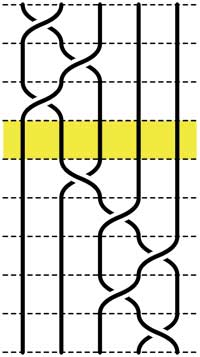Recall that an n-braid consists of n strictly descending elastic strings connecting n inputs at the top (named 1,2,…,n) to n outputs at the bottom (labeled 1,2,…,n) upto isotopy (meaning that we may pull and rearrange the strings in any way possible within 3-dimensional space). We can always change the braid slightly such that we can divide the interval between in- and output in a number of subintervals such that in each of those there is at most one crossing.

n-braids can be multiplied by putting them on top of each other and connecting the outputs of the first braid trivially to the inputs of the second. For example the 5-braid on the left can be written as $B=B_1.B_2$ with $B_1$ the braid on the top 3 subintervals and $B_2$ the braid on the lower 5 subintervals.

In this way (and using our claim that there can be at most 1 crossing in each subinterval) we can write any n-braid as a word in the generators $\sigma_i$ (with $1 \leq i < n$) being the overcrossing between inputs i and i+1. Observe that the undercrossing is then the inverse $\sigma_i^{-1}$. For example, the braid on the left corresponds to the word

$\sigma_1^{-1}.\sigma_2^{-1}.\sigma_1^{-1}.\sigma_2.\sigma_3^{-1}.\sigma_4^{-1}.\sigma_3^{-1}.\sigma_4$

Clearly there are relations among words in the generators. The easiest one we have already used implicitly namely that $\sigma_i.\sigma_i^{-1}$ is the trivial braid. Emil Artin proved in the 1930-ies that all such relations are consequences of two sets of ‘obvious’ relations. The first being commutation relations between crossings when the strings are far enough from each other. That is we have

$\sigma_i . \sigma_j = \sigma_j . \sigma_i$ whenever $|i-j| \geq 2$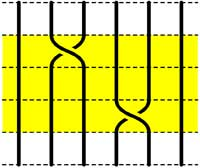=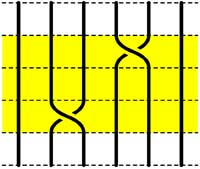The second basic set of relations involves crossings using a common string

$\sigma_i.\sigma_{i+1}.\sigma_i = \sigma_{i+1}.\sigma_i.\sigma_{i+1}$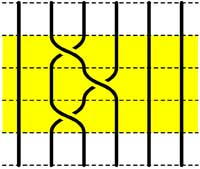=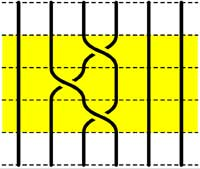Starting with the 5-braid at the top, we can use these relations to reduce it to a simpler form. At each step we have outlined to region where the relations are applied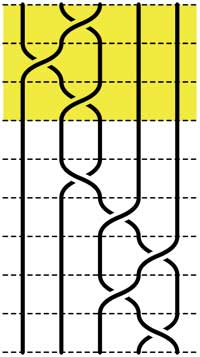=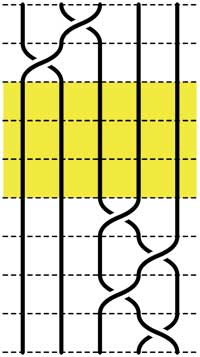=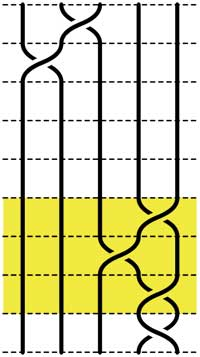=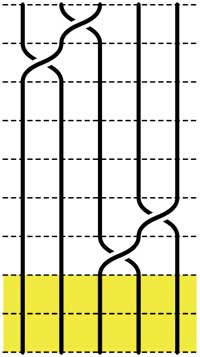These beautiful braid-pictures were produced using the braid-metapost program written by Stijn Symens.

Tracing a string from an input to an output assigns to an n-braid a permutation on n letters. In the above example, the permutation is $~(1,2,4,5,3)$. As this permutation doesn’t change under applying basic reduction, this gives a group-morphism

$\mathbb{B}_n \rightarrow S_n$

from the braid group on n strings $\mathbb{B}_n$ to the symmetric group. We have seen before that the symmetric group $S_n$ has a F-un interpretation as the linear group $GL_n(\mathbb{F}_1)$ over the field with one element. Hence, we can ask whether there is also a F-un interpretation of the n-string braid group and of the above group-morphism.

Kapranov and Smirnov suggest in their paper that the n-string braid group $\mathbb{B}_n \simeq GL_n(\mathbb{F}_1[t])$ is the general linear group over the polynomial ring $\mathbb{F}_1[t]$ over the field with one element and that the evaluation morphism (setting t=0)

$GL_n(\mathbb{F}_1[t]) \rightarrow GL_n(\mathbb{F}1)$ gives the groupmorphism $\mathbb{B}_n \rightarrow S_n$

The rationale behind this analogy is a theorem of Drinfeld‘s saying that over a finite field $\mathbb{F}_q$, the profinite completion of $GL_n(\mathbb{F}_q[t])$ is embedded in the fundamental group of the space of q-polynomials of degree n in much the same way as the n-string braid group $\mathbb{B}_n$ is the fundamental group of the space of complex polynomials of degree n without multiple roots.

And, now that we know the basics of absolute linear algebra, we can give an absolute braid-group representation

$\mathbb{B}_n = GL_n(\mathbb{F}_1[t]) \rightarrow GL_n(\mathbb{F}_{1^n})$

obtained by sending each generator $\sigma_i$ to the matrix over $\mathbb{F}_{1^n}$ (remember that $\mathbb{F}_{1^n} = (\mu_n)^{\bullet}$ where $\mu_n = \langle \epsilon_n \rangle$ are the n-th roots of unity)

$\sigma_i \mapsto \begin{bmatrix} 1_{i-1} & & & \\ & 0 & \epsilon_n & \\ & \epsilon_n^{-1} & 0 & \\ & & & 1_{n-1-i} \end{bmatrix}$

and it is easy to see that these matrices do indeed satisfy Artin’s defining relations for $\mathbb{B}_n$.

Today we will define some basic linear algebra over the absolute fields $\mathbb{F}_{1^n}$ following the Kapranov-Smirnov document. Recall from last time that $\mathbb{F}_{1^n} = \mu_n^{\bullet}$ and that a d-dimensional vectorspace over this field is a pointed set $V^{\bullet}$ where $V$ is a free $\mu_n$-set consisting of n.d elements. Note that in absolute linear algebra we are not allowed to have addition of vectors and have to define everything in terms of scalar multiplication (or if you want, the $\mu_n$-action). In the hope of keeping you awake, we will include an F-un interpretation of the power residue symbol.

Direct sums of vectorspaces are defined via $V^{\bullet} \oplus W^{\bullet} = (V \bigsqcup W)^{\bullet}$, that is, correspond to the disjoint union of free $\mu_n$-sets. Consequently we have that $dim(V^{\bullet} \oplus W^{\bullet}) = dim(V^{\bullet}) + dim(W^{\bullet})$.

For tensor-product we start with $V^{\bullet} \times W^{\bullet} = (V \times W)^{\bullet}$ the vectorspace cooresponding to the Cartesian product of free $\mu_n$-sets. If the dimensions of $V^{\bullet}$ and $W^{\bullet}$ are respectively d and e, then $V \times W$ consists of n.d.n.e elements, so is of dimension n.d.e. In order to have a sensible notion of tensor-products we have to eliminate the n-factor. We do this by identifying $~(x,y)$ with $(\epsilon_n x, \epsilon^{-1} y)$ and call the corresponding vectorspace $V^{\bullet} \otimes W^{\bullet}$. If we denote the image of $~(x,y)$ by $x \otimes w$ then the identification merely says we can pull the $\mu_n$-action through the tensor-sign, as we’d like to do. With this definition we do indeed have that $dim(V^{\bullet} \otimes W^{\bullet}) = dim(V^{\bullet}) dim(W^{\bullet})$.

Recall that any linear automorphism $A$ of an $\mathbb{F}_{1^n}$ vectorspace $V^{\bullet}$ with basis ${ b_1,\ldots,b_d }$ (representants of the different $\mu_n$-orbits) is of the form $A(b_i) = \epsilon_n^{k_i} b_{\sigma(i)}$ for some powers of the primitive n-th root of unity $\epsilon_n$ and some permutation $\sigma \in S_d$. We define the determinant $det(A) = \prod_{i=1}^d \epsilon_n^{k_i}$. One verifies that the determinant is multiplicative and independent of the choice of basis.

For example, scalar-multiplication by $\epsilon_n$ gives an automorphism on any $d$-dimensional $\mathbb{F}_{1^n}$-vectorspace $V^{\bullet}$ and the corresponding determinant clearly equals $det = \epsilon_n^d$. That is, the det-functor remembers the dimension modulo n. These mod-n features are a recurrent theme in absolute linear algebra. Another example, which will become relevant when we come to reciprocity laws :

Take $n=2$. Then, a $\mathbb{F}_{1^2}$ vectorspace $V^{\bullet}$ of dimension d is a set consisting of 2d elements $V$ equipped with a free involution. Any linear automorphism $A~:~V^{\bullet} \rightarrow V^{\bullet}$ is represented by a $d \times d$ matrix having one nonzero entry in every row and column being equal to +1 or -1. Hence, the determinant $det(A) \in \{ +1,-1 \}$.

On the other hand, by definition, the linear automorphism $A$ determines a permutation $\sigma_A \in S_{2d}$ on the 2d non-zero elements of $V^{\bullet}$. The connection between these two interpretations is that $det(A) = sgn(\sigma_A)$ the determinant gives the sign of the permutation!For a prime power $q=p^k$ with $q \equiv 1~mod(n)$, we have seen that the roots of unity $\mu_n \subset \mathbb{F}_q^*$ and hence that $\mathbb{F}_q$ is a vectorspace over $\mathbb{F}_{1^n}$. For any field-unit $a \in \mathbb{F}_q^*$ we have the power residue symbol

$\begin{pmatrix} a \\ \mathbb{F}_q \end{pmatrix}_n = a^{\frac{q-1}{n}} \in \mu_n$

On the other hand, multiplication by $a$ is a linear automorphism on the $\mathbb{F}_{1^n}$-vectorspace $\mathbb{F}_q$ and hence we can look at its F-un determinant $det(a \times)$. The F-un interpretation of a classical lemma by Gauss asserts that the power residue symbol equals $det(a \times)$.

An $\mathbb{F}_{1^n}$-subspace $W^{\bullet}$ of a vectorspace $V^{\bullet}$ is a subset $W \subset V$ consisting of full $\mu_n$-orbits. Normally, in defining a quotient space we would say that two V-vectors are equivalent when their difference belongs to W and take equivalence classes. However, in absolute linear algebra we are not allowed to take linear combinations of vectors…

The only way out is to define $~(V/W)^{\bullet}$ to correspond to the free $\mu_n$-set $~(V/W)$ obtained by identifying all elements of W with the zero-element in $V^{\bullet}$. But… this will screw-up things if we want to interpret $\mathbb{F}_q$-vectorspaces as $\mathbb{F}_{1^n}$-spaces whenever $q \equiv 1~mod(n)$.

For this reason, Kapranov and Smirnov invent the notion of an equivalence $f~:~X^{\bullet} \rightarrow Y^{\bullet}$ between $\mathbb{F}_{1^n}$-spaces to be a linear map (note that this means a set-theoretic map $X \rightarrow Y^{\bullet}$ such that the invers image of 0 consists of full $\mu_n$-orbits and is a $\mu_n$-map elsewhere) satisfying the properties that $f^{-1}(0) = 0$ and for every element $y \in Y$ we have that the number of pre-images $f^{-1}(y)$ is congruent to 1 modulo n. Observe that under an equivalence $f~:~X^{\bullet} \rightarrow Y^{\bullet}$ we have that $dim(X^{\bullet}) \equiv dim(Y^{\bullet})~mod(n)$.

This then allows us to define an exact sequence of $\mathbb{F}_{1^n}$-vectorspaces to be

$$\xymatrix{0 \ar[r] & V_1^{\bullet} \ar[r]^{\alpha} & V^{\bullet} \ar[r]^{\beta} & V_2^{\bullet} \ar[r] & 0}$$

with $\alpha$ a set-theoretic inclusion, the composition $\beta \circ \alpha$ to be the zero-map and with the additional assumption that the map induced by $\beta$

$~(V/V_1)^{\bullet} \rightarrow V_2^{\bullet}$

is an equivalence. For an exact sequence of spaces as above we have the congruence relation on their dimensions $dim(V_1)+dim(V_2) \equiv dim(V)~mod(n)$.

More importantly, if as before $q \equiv 1~mod(n)$ and we use the embedding $\mu_n \subset \mathbb{F}_q^*$ to turn usual $\mathbb{F}_q$-vectorspaces into absolute $\mathbb{F}_{1^n}$-spaces, then an ordinary exact sequence of $\mathbb{F}_q$-vectorspaces remains exact in the above definition.

All esoteric subjects have their own secret (sacred) texts. If you opened the Da Vinci Code (or even better, the original The Holy blood and the Holy grail) you will known about a mysterious collection of documents, known as the “Dossiers secrets“, deposited in the Bibliothèque nationale de France on 27 April 1967, which is rumoured to contain the mysteries of the Priory of Sion, a secret society founded in the middle ages and still active today…

The followers of F-un, for $\mathbb{F}_1$ the field of one element, have their own collection of semi-secret texts, surrounded by whispers, of which they try to decode every single line in search of enlightenment. Fortunately, you do not have to search the shelves of the Bibliotheque National in Paris, but the depths of the internet to find them as huge, bandwidth-unfriendly, scanned documents.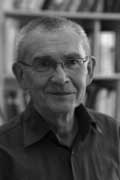The first are the lecture notes “Lectures on zeta functions and motives” by Yuri I. Manin of a course given in 1991.

One can download a scanned version of the paper from the homepage of Katia Consani as a huge 23.1 Mb file. Of F-un relevance is the first section “Absolute Motives?” in which

“…we describe a highly speculative picture of analogies between arithmetics over $\mathbb{F}_q$ and over $\mathbb{Z}$, cast in the language reminiscent of Grothendieck’s motives. We postulate the existence of a category with tensor product $\times$ whose objects correspond not only to the divisors of the Hasse-Weil zeta functions of schemes over $\mathbb{Z}$, but also to Kurokawa’s tensor divisors. This neatly leads to teh introduction of an “absolute Tate motive” $\mathbb{T}$, whose zeta function is $\frac{s-1}{2\pi}$, and whose zeroth power is “the absolute point” which is teh base for Kurokawa’s direct products. We add some speculations about the role of $\mathbb{T}$ in the “algebraic geometry over a one-element field”, and in clarifying the structure of the gamma factors at infinity.” (loc.cit. p 1-2)

I’d welcome links to material explaining this section to people knowing no motives.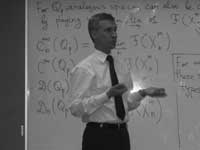The second one is the unpublished paper “Cohomology determinants and reciprocity laws : number field case” by Mikhail Kapranov and A. Smirnov.

This paper features in blog-posts at the Arcadian Functor, in John Baez’ Weekly Finds and in yesterday’s post at Noncommutative Geometry.

You can download every single page (of 15) as a separate file from here. But, in order to help spreading the Fun-gospel, I’ve made these scans into a single PDF-file which you can download as a 2.6 Mb PDF. In the introduction they say :

“First of all, it is an old idea to interpret combinatorics of finite sets as the $q \rightarrow 1$ limit of linear algebra over the finite field $\mathbb{F}_q$. This had lead to frequent consideration of the folklore object $\mathbb{F}_1$, the “field with one element”, whose vector spaces are just sets. One can postulate, of course, that $\mathbf{spec}(\mathbb{F}_1)$ is the absolute point, but the real problem is to develop non-trivial consequences of this point of view.”

They manage to deduce higher reciprocity laws in class field theory within the theory of $\mathbb{F}_1$ and its field extensions $\mathbb{F}_{1^n}$. But first, let us explain how they define linear algebra over these absolute fields.

Here is a first principle : in doing linear algebra over these fields, there is no additive structure but only scalar multiplication by field elements. So, what are vector spaces over the field with one element? Well, as scalar multiplication with 1 is just the identity map, we have that a vector space is just a set. Linear maps are just set-maps and in particular, a linear isomorphism of a vector space onto itself is a permutation of the set. That is, linear algebra over $\mathbb{F}_1$ is the same as combinatorics of (finite) sets.

A vector space over $\mathbb{F}_1$ is just a set; the dimension of such a vector space is the cardinality of the set. The general linear group $GL_n(\mathbb{F}_1)$ is the symmetric group $S_n$, the identification via permutation matrices (having exactly one 1 in every row and column)

Some people prefer to view an $\mathbb{F}_1$ vector space as a pointed set, the special element being the ‘origin’ $0$ but as $\mathbb{F}_1$ doesnt have a zero, there is also no zero-vector. Still, in later applications (such as defining exact sequences and quotient spaces) it is helpful to have an origin. So, let us denote for any set $S$ by $S^{\bullet} = S \cup { 0 }$. Clearly, linear maps between such ‘extended’ spaces must be maps of pointed sets, that is, sending $0 \rightarrow 0$.

The field with one element $\mathbb{F}_1$ has a field extension of degree n for any natural number n which we denote by $\mathbb{F}_{1^n}$ and using the above notation we will define this field as :

$\mathbb{F}_{1^n} = \mu_n^{\bullet}$ with $\mu_n$ the group of all n-th roots of unity. Note that if we choose a primitive n-th root $\epsilon_n$, then $\mu_n \simeq C_n$ is the cyclic group of order n.

Now what is a vector space over $\mathbb{F}_{1^n}$? Recall that we only demand units of the field to act by scalar multiplication, so each ‘vector’ $\vec{v}$ determines an n-set of linear dependent vectors $\epsilon_n^i \vec{v}$. In other words, any $\mathbb{F}_{1^n}$-vector space is of the form $V^{\bullet}$ with $V$ a set of which the group $\mu_n$ acts freely. Hence, $V$ has $N=d.n$ elements and there are exactly $d$ orbits for the action of $\mu_n$ by scalar multiplication. We call $d$ the dimension of the vectorspace and a basis consists in choosing one representant for every orbits. That is, $~B = { b_1,\ldots,b_d }$ is a basis if (and only if) $V = { \epsilon_n^j b_i~:~1 \leq i \leq d, 1 \leq j \leq n }$.

So, vectorspaces are free $\mu_n$-sets and hence linear maps $V^{\bullet} \rightarrow W^{\bullet}$ is a $\mu_n$-map $V \rightarrow W$. In particular, a linear isomorphism of $V$, that is an element of $GL_d(\mathbb{F}_{1^n})$ is a $\mu_n$ bijection sending any basis element $b_i \rightarrow \epsilon_n^{j(i)} b_{\sigma(i)}$ for a permutation $\sigma \in S_d$.

An $\mathbb{F}_{1^n}$-vectorspace $V^{\bullet}$ is a free $\mu_n$-set $V$ of $N=n.d$ elements. The dimension $dim_{\mathbb{F}_{1^n}}(V^{\bullet}) = d$ and the general linear group $GL_d(\mathbb{F}_{1^n})$ is the wreath product of $S_d$ with $\mu_n^{\times d}$, the identification as matrices with exactly one non-zero entry (being an n-th root of unity) in every row and every column.

This may appear as a rather sterile theory, so let us give an extremely important example, which will lead us to our second principle for developing absolute linear algebra.

Let $q=p^k$ be a prime power and let $\mathbb{F}_q$ be the finite field with $q$ elements. Assume that $q \cong 1~mod(n)$. It is well known that the group of units $\mathbb{F}_q^{\ast}$ is cyclic of order $q-1$ so by the assumption we can identify $\mu_n$ with a subgroup of $\mathbb{F}_q^{\ast}$.

Then, $\mathbb{F}_q = (\mathbb{F}_q^{\ast})^{\bullet}$ is an $\mathbb{F}_{1^n}$-vectorspace of dimension $d=\frac{q-1}{n}$. In other words, $\mathbb{F}_q$ is an $\mathbb{F}_{1^n}$-algebra. But then, any ordinary $\mathbb{F}_q$-vectorspace of dimension $e$ becomes (via restriction of scalars) an $\mathbb{F}_{1^n}$-vector space of dimension $\frac{e(q-1)}{n}$.

Next time we will introduce more linear algebra definitions (including determinants, exact sequences, direct sums and tensor products) in the realm the absolute fields $\mathbb{F}_{1^n}$ and remarkt that we have to alter the known definitions as we can only use the scalar-multiplication. To guide us, we have the second principle : all traditional results of linear algebra over $\mathbb{F}_q$ must be recovered from the new definitions under the vector-space identification $\mathbb{F}_q = (\mathbb{F}_q^{\ast})^{\bullet} = \mathbb{F}_{1^n}$ when $n=q-1$. (to be continued)

At the
noncommutative algebra program in MSRI 1999/2000, Mikhail Kapranov gave
an intriguing talk Noncommutative neighborhoods and noncommutative Fourier transform
and over the years I’ve watched the video of this talk a number
of times. The first part of the talk is about his work on Noncommutative geometry
based on commutator expansions
and as I’ve once worked through it
this part didn’t present problems. On the other hand, I’ve never
understood much from the second part of the talk which claims to relate
these noncommutative formal neighborhoods to _noncommutative Fourier
transforms_. The string coffee table has a post Kapranov
and Getzler on Higher Stuff
linking to two recent talks by Kapranov
on noncommutative Fourier transforms at the Streetfest. Marni
Sheppeard made handwritten notes available. I definitely should find the time
to get through them and have another go at the Kapranov-video…

Now that the preparation for my undergraduate courses in the first semester is more or less finished, I can begin to think about the courses I’ll give this year in the master class
non-commutative geometry. For a change I’d like to introduce the main ideas and concepts by a very concrete example : Ginzburg’s coadjoint-orbit result for the Calogero-Moser space and its
relation to the classification of one-sided ideals in the first Weyl algebra. Not only will this example give me the opportunity to say things about formally smooth algebras, non-commutative
differential forms and even non-commutative symplectic geometry, but it also involves what some people prefer to call _non-commutative algebraic geometry_ (that is the study of graded Noetherian
rings having excellent homological properties) via the projective space associated to the homogenized Weyl algebra. Besides, I have some affinity with this example.

A long time ago I introduced
the moduli spaces for one-sided ideals in the Weyl algebra in Moduli spaces for right ideals of the Weyl algebra and when I was printing a _very_ preliminary version of Ginzburg’s paper
Non-commutative Symplectic Geometry, Quiver varieties, and Operads (probably because he send a preview to Yuri Berest and I was in contact with him at the time about the moduli spaces) the
idea hit me at the printer that the right way to look at the propblem was to consider the quiver

$\xymatrix{\vtx{} \ar@/^/[rr]^a & & \vtx{} \ar@(u,ur)^x \ar@(d,dr)_y \ar@/^/[ll]^b}$

which eventually led to my paper together with Raf Bocklandt Necklace Lie algebras and noncommutative symplectic geometry.

Apart from this papers I would like to explain the following
papers by illustrating them on the above example : Michail Kapranov Noncommutative geometry based on commutator expansions Maxim Kontsevich and Alex Rosenberg Noncommutative smooth
spaces
Yuri Berest and George Wilson Automorphisms and Ideals of the Weyl Algebra Yuri Berest and George Wilson Ideal Classes of the Weyl Algebra and Noncommutative Projective
Geometry
Travis Schedler A Hopf algebra quantizing a necklace Lie algebra canonically associated to a quiver and of course the seminal paper by Joachim Cuntz and Daniel Quillen on
quasi-free algebras and their non-commutative differential forms which, unfortunately, in not available online.

I plan to write a series of posts here on all this material but I will be very